# GATE Virtual Calculator - Explore How to Use

GATE Virtual Calculator – Candidates appearing for the GATE 2024 Exam are not allowed to take certain items into the hall, and the calculator is one such product. However, candidates are permitted to access the GATE virtual calculator. Now, the question arises, what is a virtual scientific calculator? Since most of the questions asked in the GATE exam are numerical-based, a calculator is required to answer them. For this reason, the exam authorities provide access to a unique feature to the candidates, the GATE virtual calculator for PC. This feature has been made available to GATE aspirants since 2016.

However, since this virtual calculator in the GATE exam is a complicated device, one must know how to use it. Keeping this in mind, in this article, we have given the complete details about the virtual calculator for GATE download for PC and how to use it.

## Specifications of GATE Virtual Calculator

To understand what a GATE scientific calculator is or how to use it properly, the candidate should first need to know the basic features of the GATE calculator for PC.

• Candidates who appear for the GATE exams will be allowed by the authorities at the exam centre to access the virtual calculator in the GATE exam.
• The keys to operate simple functions can be found on the right side of the GATE virtual calculator.
• For scientific operations or other functions, the keys will be available on the left side of the GATE virtual calculator.
• Since there are separate keys for inverse functions, the candidates are not required to click on the SHIFT or INV keys.
• Inverse functions are below the respective functions. Given here is the example where ‘sin’ is now available; sin-1 can be found below, as per the calculator.
• All the trigonometric functions can be found grouped.
• Hyperbolic functions are also grouped in the virtual calculator.
• Two display boxes are shown at the top of the virtual calculator, where one package depicts the sequence of the keys that the candidate presses for reference.
• Another box shows the actual values that are entered or obtained after the completion of the calculations.
• Here, we have provided screenshots of at least one calculation for each operation.

### Given below are the examples for these calculations:

1. Enter ‘C’ to clear all inputs.
2. Enter (←) to delete the last digit entered in the input.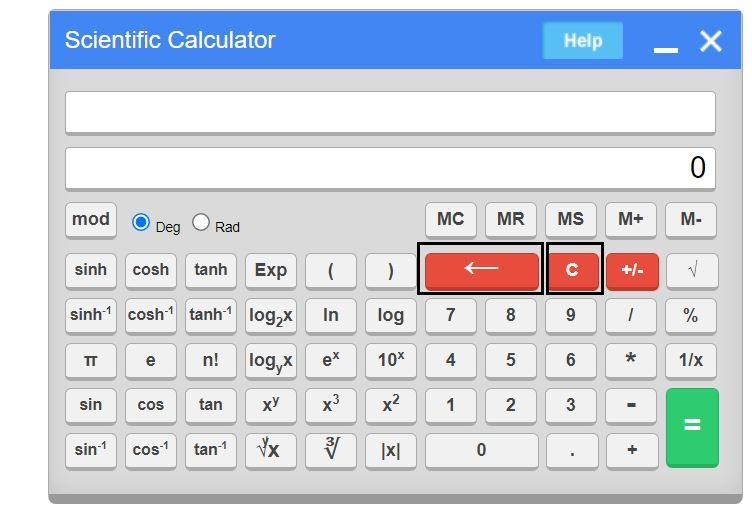• How do we calculate simple arithmetic calculations?
1. To calculate 60 + 35 x 5, candidates have to enter 60, +, 35, *, 5, =
2. To evaluate: 75 x 40-3, candidates should enter 75, *, 40, -, 3, =
3. Also, to evaluate: 73 x (2+3), candidates have to enter 73, *, (, 2, +, 3, ), =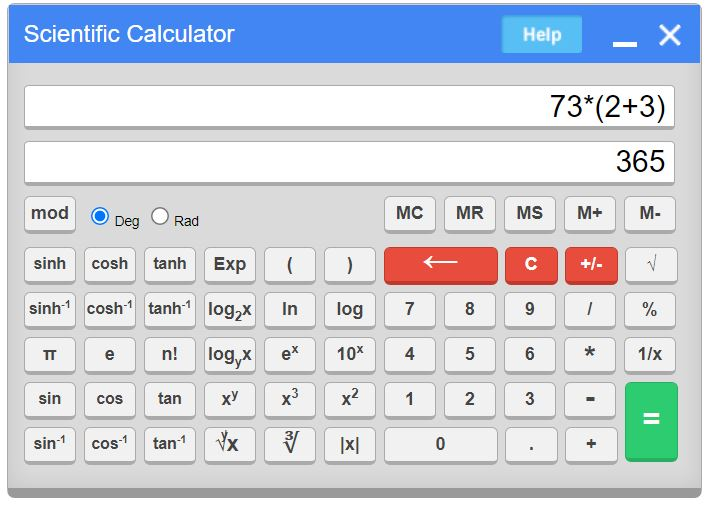• How do we evaluate function value?
1. To calculate 2 + log(30) x 10, candidates should enter 30, log, *, 10, +, 2, =
2. To calculate log(20) x 3 – 7, candidates have to enter 20, log,*, 3, -, 7, =
3. To calculate In(15) x 2 + 8, candidates should enter 15, In, *, 2, +, 8, =
4. To evaluate 3 + In(15) x 2, candidates have to enter 15, In, *, 2, +, 3,=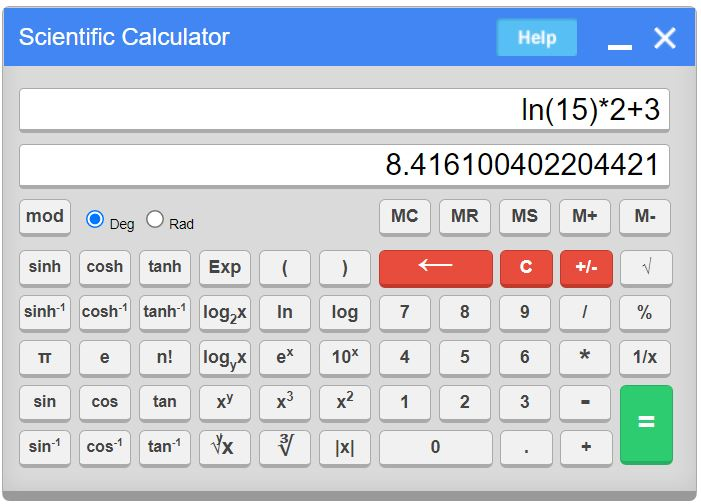• How do we use sin(Degree), cos(Degree), tan(Degree)?
1. To calculate sin(25) or cos(25) or tan(25), candidates must enter 25, sin or cos or tan
2. To evaluate: tan(35) x 6 + 2, candidates must enter 35, tan, *, 6, +, 2, =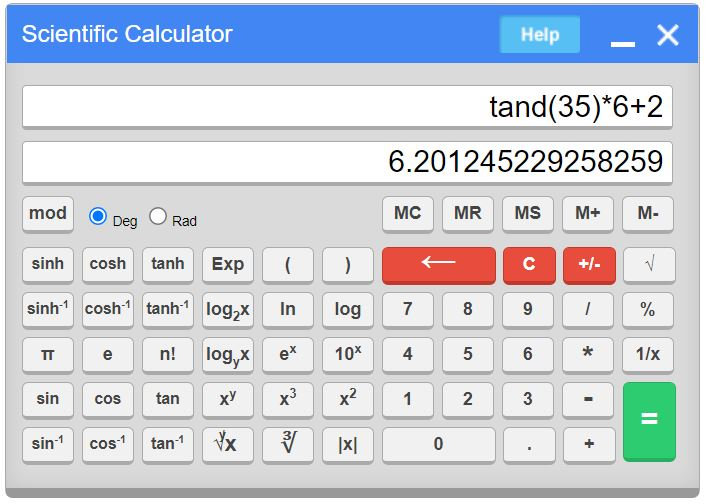• How to find out the yroot() value and cube() value?
1. To evaluate the 4th root of 8, candidates are required to enter 8, , 4, =
2. To evaluate: 483 x 6+9, candidates are all asked to enter 48, x3, *, 6, +, 9, =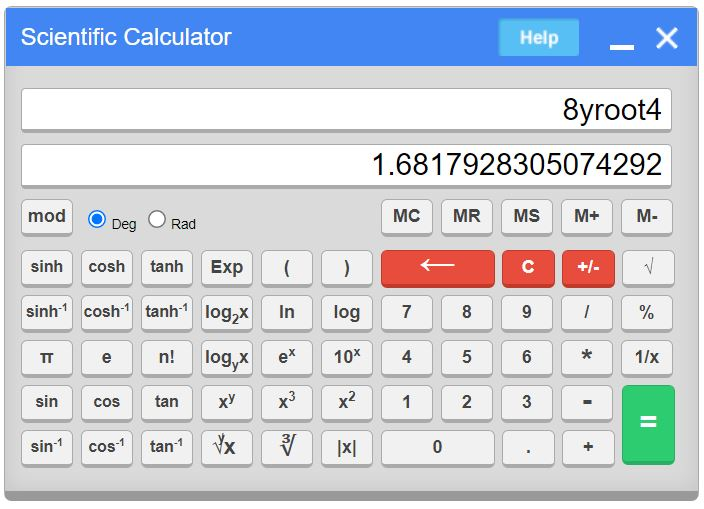• How to find the value of cube root() and square root?
1. To evaluate cube root(5), candidates must enter 5, .
2. To evaluate square root(6), candidates must enter 6, .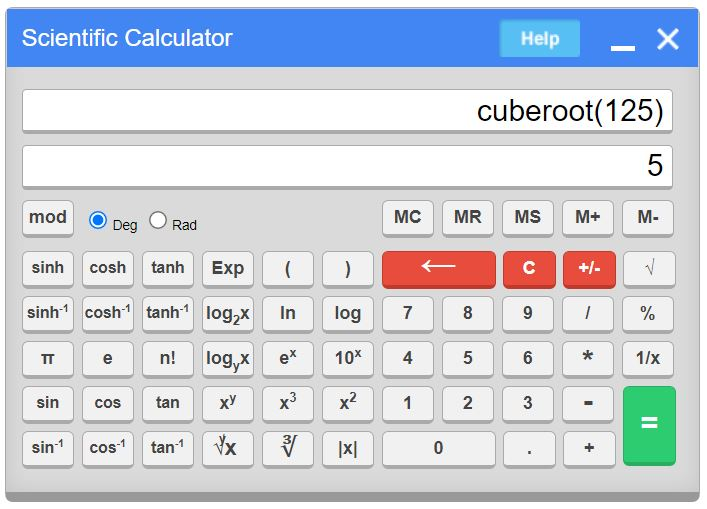• How to find out the factorial (n!) and exponential (e)?
1. To evaluate factorial(5), candidates must enter 5, n!
2. To evaluate factorial(8)*5-2, candidates must enter 8, n!, *, 5, -, 2, =
3. To evaluate e0, candidates must enter 0, ex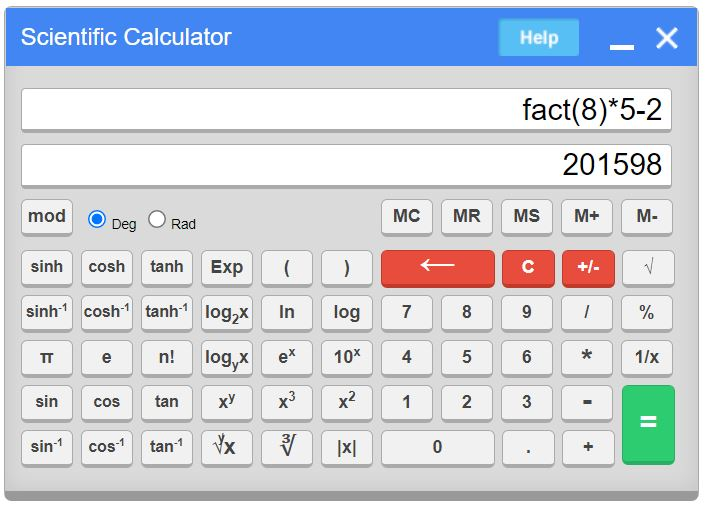• How do we find xy?
1. To evaluate 18-0.509, candidates must enter 18, xy, 0.509, +/-, =
2. To evaluate 20-2, candidates must enter 20, xy, 2, +/-, =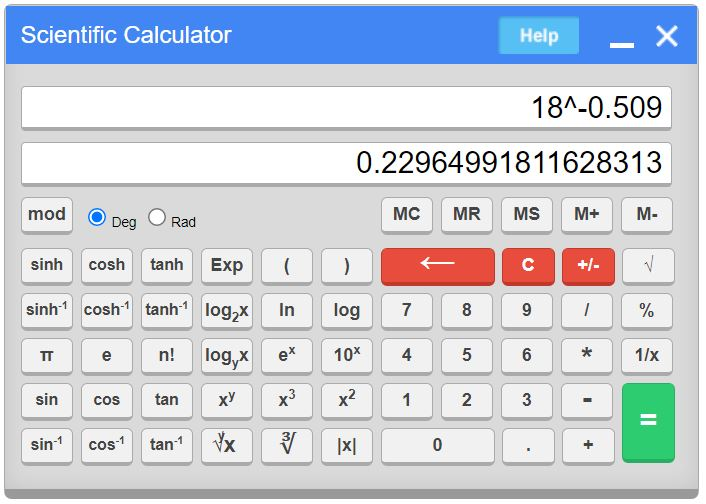### Tips to Use GATE Virtual Calculator Effectively

We have listed a few tips and tricks to use the GATE virtual calculator to solve problems as soon as possible. This can help candidates to save time and use it for effective calculation in the exam. Candidates can apply the following effective tips on the GATE calculator during GATE 2024 exam.

• Press [C] at the start of a calculation to clear any previously calculated value
• Usage of the predefined operators can help in fast calculations. For example, π, log, ln, n!
• Try to put brackets for the complex operators to avoid wrong calculations
• Use the memory keys for lengthy calculations

### Memory Keys (MC, MR, MS, M+, M-) in GATE Calculator

• Press MC to clear the memory
• Press MS to store the number in memory
• Press MR to read the number from the memory
• Press M+ to add the memory to the number available on display, then store it in memory
• Press M- to subtract the number on display from the memory value and then store it in memory

For any further details about the GATE Exam Pattern and the other exam resources, candidates can stay tuned to BYJU’S.